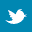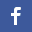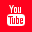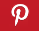Completely Solved C, C++ Programs Assignment.Quick Search Database Videos Tutorials Ebooks Forums FAQ Aboutus Household Industrial Manufacturing Service Shopping Transportation### C program to implement Dijkstra's algorithm to find the shortest cost (also shortest path) between two specific vertices of a weighted graph.

 Filed Under: C Assignments

Program Statement :
Write a C program to implement Dijkstra's algorithm to find the shortest cost (also shortest path) between two specific vertices of a weighted graph.

Theory :
Dijkstra's algorithm is used to solve the single source shortest path problem of a weighted graph. There is a source vertex vs and a destination vertex vz which are given as input by the user. The strategy is to start from vs and systematically build up a list of shortest paths to all vertices that lie between vs and vz until vz is reached. A weighted graph is taken as input in the form of a vertex by vertex matrix such that (i) w(i,j) = 0 if vertices vi and vj has no direct path between them and
(ii) if vi and vj are directly connected by an edge then w(i,j) = weight of that edge.
This algorithm requires three arrays:
(i) length[] = array of distances
(ii) set[] = array of Boolean tags
(iii) path[] = array of vertices.
In conclusion, the shortest distance from the source vertex to any vertex (which lie between vs and vz) is stored in length[]. Path[] contains the nearest predecessor(s) of vz. In other words, path[] keeps a track of the shortest path from vz to vs. At each iteration, when a particular vertex (say vi) with minimum label is selected that vertex will be included in set[] i.e set[i] will be changed to 1 from 0. After the executions of the algorithm, we get the shortest cost from length[vz] and to get the shortest path we must back track through path[].

GRAPH G

Algorithm :
/* The input graph, no. of vertices n, source vertex vs and destination vertex vz are passed as parameters */
Algo_dijkstra(a[][], n, vs, vz)
{
for(i=1 to n)
set[i] = 0;
for(i=1 to n)
{
if(a[vs][i] = 0) /* There is no direct edge between vs and vi */
{
length[i] = infinity;
path[i] = 0; /* Empty path */
}
else
{
length[i] = a[vs][i];
path[i] = vs; /* vs is immediate predecessor of vi */
}
}
set[vs] = 1; /* vs is included in the set */
length[vs] = 0; /* vs is implicitly enumerated with length as 0 */
while(vz is not included in the set[])
{
/* Select a vertex vj with minimum label such that it is not included in the set[]*/
j=srch_min(length,set,n);
set[j]=1; /* vj is included in the set[] */
for(i=1 to n)
{
if(vi is not already in set[])
{
if(vi and vj is connected by an edge)
{
/*When exsisting label is not minimum only then replacement is done*/
if(length[j]+a[i][j]<length[i])
{
length[i] = length[j]+a[i][j];
path[i]=j;
}
}
}
}
}
cost = length[vz];
print(cost); /* Print the shortest cost */
print(path[]); /* Print the shortest path by back tracking */
}/* End of Algo_dijkstra */

Program listing :
/* C program to implement Dijkstra's algorithm to find shortest cost between any vertex pair */
#include<stdio.h>
#define inf 9999
#define size 10/*Defining maximum size of the matrix*/
main()
{
int a[size][size],i,j,n,v1,v2,lcost;
int dij(int[][j],int,int,int);
printf("Enter the number of vertex : ");
scanf("%d",&n);
/*Input 0 if there is no direct edge between vertex pair*/
printf("Enter a weighted matrix(with weights) as input :n");
for(i=0;i<n;i++)
{
for(j=0;j<n;j++)
{
printf("Enter the value of a[%d][%d] : ",i,j);
scanf("%d",&a[i][j]);
}
}
printf("The entered matrix is:n");
for(i=0;i<n;i++)
{
for(j=0;j<n;j++)
printf("%dt",a[i][j]);
printf("n");
}
printf("Enter starting vertex v");
scanf("%d",&v1);
printf("Enter ending vertex v");
scanf("%d",&v2);
/*Check for validity of input vertices*/
if(v1<0||v1>n-1||v2<0||v2>n-1)
{
printf("!!!!!ERROR!!!!!n");
printf("!!!!!Invalid vertex given!!!!!");
return;
}
printf("Shortest path between v%d & v%d : ",v1,v2);
lcost=dij(a,n,v1,v2);
printf("Shortest cost between v%d & v%d : ",v1,v2);
printf("%d",lcost);/*Print the shortest cost*/
}
/*The input graph,no. of vertices n,source vertex v1 and destination vertex v2 are passed as parameters*/
int dij(int a[size][size],int n,int v1,int v2)
{
int length[size],set[size],path[size],i,j,s,z,tmp,temp[size],c=0,f=0;
s=v1;
z=v2;
int srch_min(int[],int[],int);
for(i=0;i<n;i++)
set[i]=0;
for(i=0;i<n;i++)
{
if(a[s][i]==0)/*There is no direct edge between vertices s and i*/
{
length[i]=inf;
path[i]=0;/*Empty path*/
}
else
{
length[i]=a[s][i];
path[i]=s;/*s is immediate predecessor of i*/
}
}
set[s]=1;/*s is included in the set*/
length[s]=0;/*s is implicitly enumerated with length as 0*/
while(set[z]!=1)/*Iteration will be considered until final vertex z belongs to s*/
{
j=srch_min(length,set,n);/*Select a vertex j with minimum label such that it is not included in the set[]*/
set[j]=1;/*Vertex j is included in the set[]*/
for(i=0;i<n;i++)
{
if(set[i]!=1)
{
if(a[i][j]!=0)
{
if(length[j]+a[i][j]<length[i])/*When exsisting label is not minimum only then replacement is done*/
{
length[i]=length[j]+a[i][j];
path[i]=j;
}
}
}
}
}
j=0;
i=z;
while(i!=s)
{
tmp=path[i];
temp[j]=tmp;
i=tmp;
j++;
c++;
}
for(j=c-1;j>=0;j--)
{
printf("v%d->",temp[j]);/*Print the shortest path*/
if(temp[j]==z)
f=1;
}
if(f!=1)
printf("v%d",z);
printf("n");
return length[z];
}
/*This function will return a vertex with minimum label such that it is not included in set[]*/
int srch_min(int length[],int set[],int n)
{
int min,i,min_index;
min=99999,min_index;
for(i=1;i<n;i++)
{
if(set[i]!=1)
{
if(length[i]<min)
{
min=length[i];
min_index=i;
}
}
}
return min_index;
}

Output : ( FOR GRAPH G)
Enter the number of vertex : 5
Enter a weighted matrix(with weights) as input :

Enter the value of a : 0
Enter the value of a : 2
Enter the value of a : 1
Enter the value of a : 5
Enter the value of a : 0
Enter the value of a : 2
Enter the value of a : 0
Enter the value of a : 0
Enter the value of a : 1
Enter the value of a : 3
Enter the value of a : 1
Enter the value of a : 0
Enter the value of a : 0
Enter the value of a : 1
Enter the value of a : 0
Enter the value of a : 5
Enter the value of a : 1
Enter the value of a : 1
Enter the value of a : 0
Enter the value of a : 2
Enter the value of a : 0
Enter the value of a : 3
Enter the value of a : 0
Enter the value of a : 2
Enter the value of a : 0

The entered matrix is:

0 2 1 5 0
2 0 0 1 3
1 0 0 1 0
5 1 1 0 2
0 3 0 2 0

Enter starting vertex v0
Enter ending vertex v4

Shortest path between v0 & v4 : v0->v2->v3->v4

Shortest cost between v0 & v4 : 4

Discussions :
● The worst case time complexity of Dijkstra's algorithm is O( n2 ), where n is the number of vertices.
● For sparse graphs, that is, graphs with far fewer than O( | V | 2) edges, Dijkstra's algorithm can be implemented more efficiently by storing the graph in the form of adjacency lists and using a binary heap, pairing heap, or Fibonacci heap as a priority queue to implement extracting minimum efficiently. With a binary heap, the algorithm requires O(( | E | + | V | )log | V | ) time (which is dominated by O( | E | log | V | ), assuming the graph is connected), and the Fibonacci heap improves this to O( | E | + | V | log | V | ), where | E | is the number of edges of the graph and | V | is the number of vertices of the graph.
● The weights of the edges of the graph should be non-negative real numbers else the algorithm will not work.
● The infinity value is taken as 9999 as input.
● The source and destination vertices should not be less than zero or greater than (n-1), where n is the number of vertices otherwise the program will not work properlyBack to main directory:Software Practical